# Samacheer Kalvi 11th Economics Solutions Chapter 4 Cost and Revenue Analysis

Guys who are planning to learn the fundamentals of Economics Solutions can avail the handy study material Samacheer Kalvi Class 11th Economics Solutions Chapter 4 Cost and Revenue Analysis Questions and Answers here. Refer to the Samacheer Kalvi Economics Solutions for Class 11th PDF available and score better grades. Simply click on the quick links available for Samacheer Kalvi 11th Economics Solutions Chapter 4 Cost and Revenue Analysis and prepare all the concepts in it effectively. Take your preparation to the next level by availing the Tamilnadu State Board Solutions for Class 11th Economics Solutions Chapter 4 Cost and Revenue Analysis prepared by subject experts.

## Tamilnadu Samacheer Kalvi 11th Economics Solutions Chapter 4 Cost and Revenue Analysis

Do you feel the concept of Economics Solutions difficult to understand? Not anymore with our Samacheer Kalvi for Class 11th Economics Solutions Chapter 4 Cost and Revenue Analysis Questions and Answers. All the Solutions are given to you with detailed explanation and you can enhance your subject knowledge. Use the Samacheer Kalvi Textbook Chapter 4 Cost and Revenue Analysis Solutions PDF free of cost and prepare whenever you want.

### Samacheer Kalvi 11th Economics Cost and Revenue Analysis Text Book Back Questions and Answers

Part – A

Multiple Choice Questions

Question 1.
Cost refers to _______
(a) price
(b) value
(c) fixed cost
(d) cost of production
(d) cost of production

Question 2.
Cost functions are also known as …………………….. function.
(a) Production
(b) Investment
(c) Demand
(d) Consumption
(a) Production

Question 3.
Money cost is also known as _______ cost.
(a) explicit
(b) implicit
(c) social
(d) real
(a) explicit

Question 4.
Explicit cost plus implicit cost denote ………………… cost.
(a) Social
(b) Economic
(c) Money
(d) Fixed
(b) Economic

Question 5.
Explicit costs are termed as
(a) out of pocket expenses
(b) social cost
(c) real cost
(d) sunk cost
(a) out of pocket expenses

Question 6.
The costs of self – owned resources are termed as ……………………… cost.
(a) Real
(b) Explicit
(c) Money
(d) Implicit
(d) Implicit

Question 7.
The cost that remains constant at all levels of output is _______ cost.
(a) fixed
(b) variable
(c) real
(d) social
(a) fixed

Question 8.
Identify the formula for estimating average variable cost.
(a) TC/Q
(b) TVC/Q
(c) TFC/Q
(d) TAC/Q
(b) TVC/Q

Question 9.
The cost incurred by producing one more unit of output is _______ cost.
(a) variable
(b) fixed
(c) marginal
(d) total
(c) marginal

Question 10.
The cost that varies with the level of output is termed as …………………. cost.
(a) Money
(b) Variable cost
(c) Total cost
(d) Fixed cost
(b) Variable cost

Question 11.
Wage is an example for _______ cost of the production.
(a) fixed
(b) variable
(c) marginal
(d) opportunity
(b) variable

Question 12.
The cost per unit of output is denoted by …………………… cost.
(a) Average
(b) Marginal
(c) Variable
(d) Total
(a) Average

Question 13.
Identify the formula of estimating average cost.
(a) AVC/Q
(b) TC/Q
(c) TVC/Q
(d) AFC/Q
(b) TC/Q

Question 14.
Final total cost where TFC = 100 and TVC = 125.
(a) 125
(b) 175
(c) 225
(d) 325
(c) 225

Question 15.
Long-run average cost curve is also called as _______ curve.
(a) demand
(b) planning
(c) production
(d) sales
(b) planning

Question 16.
Revenue received from the sale of products is known as …………………….. revenue.
(a) Profit
(b) Total revenue
(c) Average
(d) Marginal
(b) Total revenue

Question 17.
Revenue received from the sale of an additional unit is termed as _______ revenue.
(a) profit
(b) average
(c) marginal
(d) total
(c) marginal

Question 18.
(a) Total sales
(b) Total revenue
(c) Total production
(d) Total cost
(b) Total revenue

Question 19.
When price remains constant, AR will be _______ MR.
(a) equal to
(b) greater than
(c) less than
(d) not related to
(a) equal to

Question 20.
A bookseller sold 40 books with a price of ₹10 each. The total revenue of the sellers is ₹ …………………….
(a) 100
(b) 200
(c) 300
(d) 400
(d) 400

Part – B

Answer the following questions in one or two sentences

Question 21.
Define cost.
Cost refers to the total expenses incurred in the production of a commodity.

Question 22.
Define cost function?
The functional relationship between cost and output is expressed as ‘Cost Function’.
A Cost Function may be written as
C = f (Q)
Eg: TC = Q3 – 18Q2 + 91Q + 12
Where, C = Cost, and Q = quantity of output. Cost functions are derived functions because they are derived from Production Functions.

Question 23.
What do you mean by fixed cost?
Fixed cost does not change with the change in the quantity of output. The expenses on fixed factors remain unchanged irrespective of the level of output and these expenses are called fixed cost.

Question 24.
Define Revenue?
The amount of money that a producer receives in exchange for the sale of goods is known as revenue. In short, revenue means sales revenue. It is the amount received by a firm from the sale of a given quantity of a commodity at the prevailing price in the market.

Question 25.
Explicit Cost – Define.
Explicit cost refers to the actual expenditures of the firm to purchase or hire the inputs.

Question 26.
Give the definition for ‘Real Cost’?
Real Cost refers to the payment made to compensate for the efforts and sacrifices of all factor owners for their services in production. Real Cost includes the efforts and sacrifices of landlords in the use of land, capitalists to save and invest, and workers in foregoing leisure. Real costs are considered pains and sacrifices of labour as the real cost of production.

Question 27.
What is meant by Sunk cost?
A cost incurred in the past and cannot be recovered in the future is called a sunk cost.

Part – C

Answer the following questions in One Paragraph

Question 28.
Distinguish between fixed cost and variable cost.
Fixed Cost:

1. Does not change with the change in the quantity of output.
2. It is also called as ‘Supplementary cost’ or ‘Overhead cost’.
(Eg.) Rent of the factory, Permanent worker’s salary.

Variable Cost:

1. These costs vary with the level of output
2. It is also called as ‘Prime cost’, ‘Special cost’ or ‘Direct cost’.
(Eg.) Cost of raw materials, Temporary worker’s salary.

Question 29.
State the differences between money cost and real cost.
Money Cost:

1. It is the total money expenses incurred by a firm in producing a commodity.
2. It includes the cost of raw materials, payments of wages and salaries, rent, interest, etc.,
3. It is also called as prime cost or direct cost or nominal cost.

Real Cost:

1. It refers to the payment made to compensate for the efforts and sacrifices of all factor owners for their services in production.
2. It includes the efforts and sacrifices of factors of production.
3. Landlords effort is the use of land, capitalists to save and invest and workers in foregoing leisure.

Question 30.
Distinguish between explicit cost and implicit cost.
Explicit Cost:

1. Payment made to others for the purchase of factors of production.
2. It includes wages, payment for raw material, rent, interest, expenditure on transport and advertisement.
3. It is also called as accounting cost or out of pocket cost or money cost.

Implicit Cost:

1. Payment made to the use of resources that the firm already owns.
2. Cash payment is not made for the use of producer’s own land, building, machinery and other factors of production.
3. Implicit cost is also called as imputed cost or book cost.

Question 31.
Define opportunity cost and provide an example?

1. Opportunity cost refers to the cost of the next best alternative use. In other words, it is the value of the next best alternative foregone.
2. For example, a farmer can cultivate both paddy and sugarcane in farmland.
3. If he cultivates paddy, the opportunity cost of paddy output is the amount of sugarcane output given up.
4. Opportunity Cost is also called as “Alternative Cost” or Transfer cost.

Question 32.
Stale the relationship between AC and MC.
There is a unique relationship between the AC and MC curves.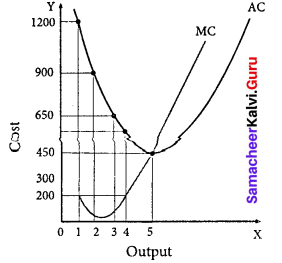1. When AC is falling, MC lies below AC.
2. When AC becomes constant, MC also becomes equal to it.
3. When AC starts increasing, MC lies above the AC.
4. MC curve always cuts AC at its minimum point from below.

Question 33.
Write a short note on Marginal Revenue?

1. Marginal Revenue [MR] is the addition to the total revenue by the sale of an additional unit of a commodity.
2. MR can be found out by dividing change in total revenue by the change in quantity sold out.
3. MR = ∆TR/∆Q where MR denotes Marginal Revenue, ∆TR denotes change in Total Revenue and ∆Q denotes change in total quantity.
4. The other method of estimating MR is:

MR = TRn – TRn-1, (or) TRn+1 – TRn
Where, MR denotes Marginal Revenue,
TRn denotes total revenue of nth item,
TRn-1 denotes Total Revenue of n – 1th item and
TRn+1 denotes Total Revenue of n + 1th item.
If TR = PQ,
MR = dTR/dQ = P, which is equal to AR.

Question 34.
Discuss the Long run cost curves with a suitable diagram.
In the long run all factors of production become variable. 1 he existing size of the firm can be increased. There are neither fixed inputs nor fixed costs in the long run.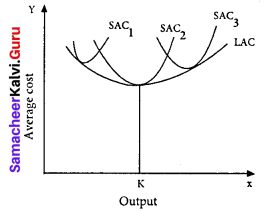LAC = LTC/Q
LAC – Long-run average cost LTC – Long-run total cost
Q – denotes the quantity of output.
The LAC curve is derived from short run average cost curves. It is the locus of points denoting the least cost curve of producing the corresponding output.
The LAC curve is called as ‘Planning curve’ or ‘Envelope curve’

Part – D

Question 35.
If total cost =10+Q3, find out AC, AVC, TFC, AFC when Q = 5.
TC = 10 + Q3
AC = $$\frac { TC }{ Q }$$
AC = $$\frac{10+Q^{3}}{Q}$$
If Q=5, Q = 5 × 5 × 5 = 125
AC = $$\frac { 10 + 125 }{ 5 }$$ = $$\frac { 135 }{ 5 }$$ = 27

AVC:
TC = 10 + Q3
TC = TFC + TVC
TVC = Q3
AVC = $$\frac { TVC }{ Q }$$
= $$\frac{Q^{3}}{Q} = Q$$2
If Q = 5, then AVC = 52
AVC = 25

TFC:
TC = 10 + Q3
TC = TFC + TVC
TFC = 10

AFC:
AFC = $$\frac { TFC }{ Q }$$
= $$\frac { 10 }{ 5 }$$
AFC = 2

Question 36.
Discuss the short run cost curves with suitable diagram :
1. Total fixed cost: TFC
All payments for the fixed factors of production are known as total fixed cost. It does not change with output.

2. Total variable cost: TVC
All payments to the variable factors of production is called as total variable cost. As output increases TVC also increases.

3. Total cost curves: TC
Total cost means the sum total of all payments made in the production. It is the total cost of production.
TC = TFC + TVC.

4. Average fixed cost: AFC
It refers to the fixed cost per unit of output.
AFC = $$\frac { TFC }{ Q }$$

5. Average variable cost: AVC
It refers to the total variable cost per unit of output.
AVC = $$\frac { TFC }{ Q }$$

6. Average cost:
It refers to the total cost per unit of output.
AC = $$\frac { TC }{ Q }$$ (or) AC = AFC + AVC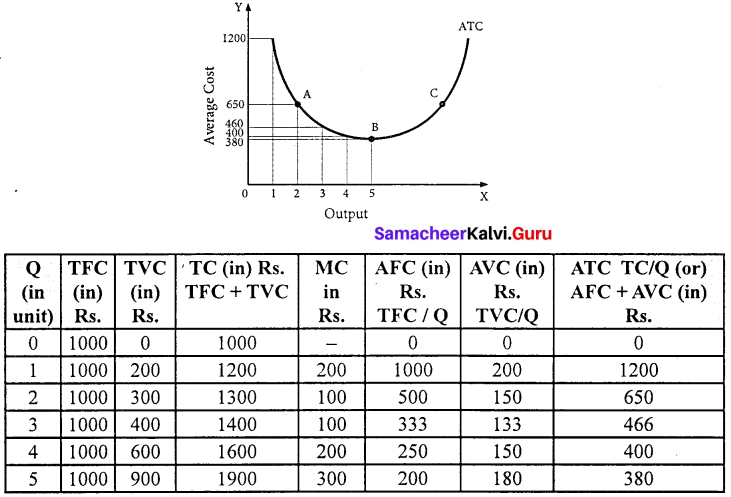1. ATC curve is also a ‘U’ shaped curve.
2. Initially ATC declines, reaches a minimum and rises beyond the optimum output.
3. The ‘U’ shape of the AC reflects the law of the variable proportions.

Marginal Cost:
Marginal cost is the additional made to the total cost by producer one extra unit of output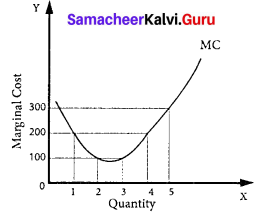Question 37.
Bring out the relationship between AR and MR curves under various price conditions.
If a firm is able to sell additional units at the same price then AR and MR will be constant. If the firm sells its additional units only by reducing the price then both AR and MR will fall and be different.

Constant AR and MR: (at fixed price)
If price remains constant, MR also remain constant and coincide with AR. Under perfect competition as the price is constant, AR is equal to MR and their shape will be straight line horizontal to X axis.
TR – AR – MR – Constant price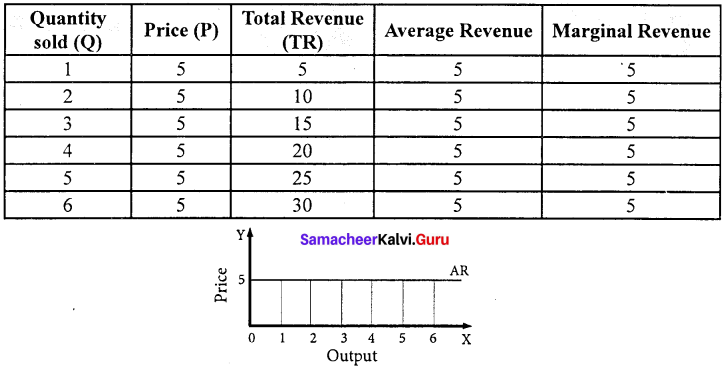Declining AR and MR: (at declining price)

When a firm sells large quantities at lower prices both AR and MR will fall. But the fall in MR will be more steeper than the fall in the AR and MR lies below AR.

The MR curve divides the distance between AR curve and Y axis into two equal parts. The decline in AR need not be a straight line or linear. If the prices are declining with the increase in quantity sold, the AR can be non-linear may be concave or convex to the origin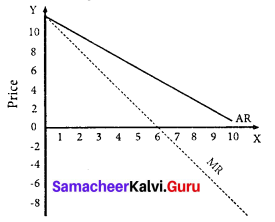AR,TR,MR declining price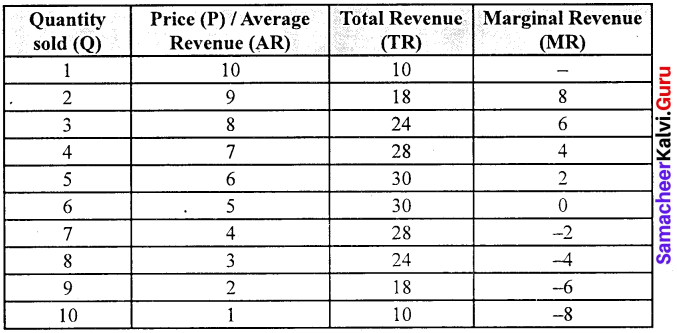### Samacheer Kalvi 11th Economics Cost and Revenue Analysis Additional Questions and Answers

Part – A

Choose the best options

Question 1.
Real Costis ……………………
(a) Pain and sacrifice
(b) Subjective concept
(c) Efforts and foregoing leisure
(d) All the above
(d) All the above

Question 2.
Average fixed cost is obtained by _______
(a) TC / Q
(b) TFC / Q
(c) TVC / Q
(d) None of the above
(b) TFC / Q

Question 3.
The Marginal Cost curve is ……………………..
(a) V-shaped
(b) Upward
(c) Downward
(d) U – shaped
(d) U – shaped

Question 4.
Total fixed cost + Total variable cost is?
(a) AC-MC
(b) TC-AC
(c) TC
(d) None
(c) TC

Question 5.
What is break-even point?
(a) No profit no loss point
(b) No profit
(c) No loss
(d) Profit – point
(a) No profit no loss point

Question 6.
Break-Even point is _______
(a) Total cost and total revenue
(b) Average revenue and financial revenue
(c) No profit – no loss point
(d) All the above
(c) No profit – no loss point

Question 7.
What is the other name for “Opportunity Cost”?
(a) Real Cost
(b) Money Cost
(c) Economic Cost
(d) Social Cost
(a) Real Cost

Question 8.
Average variable cost is _______
(a) TFC / Q
(b) TVC / Q
(c) TC / Q
(d) None
(b) TVC / Q

Question 9.
…………………….. revenue means price of the product.
(a) Total
(b) Marginal
(c) Profit
(d) Average
(d) Average

Question 10.
Cost function is the
(a) Relationship between total cost and output
(b) Relationship between revenue and cost
(c) Relationship between wages and interest d. None of the above
(d) None of this above
(a) Relationship between total cost and output

Question 11.
Match the following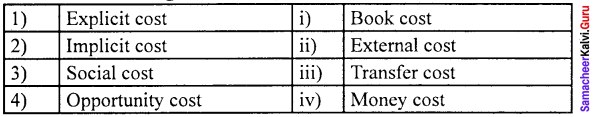(a) 1 – (iv), 2 – (i), 3 – (iii), 4 – (ii)
(b) 1 – (iv), 2 – (i), 3 – (ii), 4 – (iii)
(c) 1 – (ii), 2 – (iii), 3 – (iv), 4 – (i)
(d) 1 – (iii), 2 – (iv), 3 – (i), 4 – (ii)
(b) 1 – (iv), 2 – (i), 3 – (ii), 4 – (iii)

Match the following and choose the answer using the codes given below

Question 1.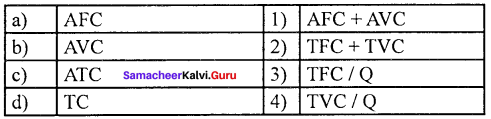(a) 1 2 3 4
(b) 3 4 1 2
(c) 2 3 4 1
(d) 4 3 1 2
(b) 3 4 1 2

Question 2.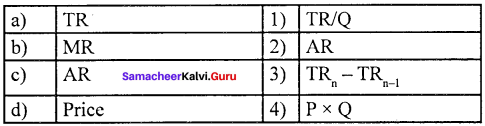(a) 3 4 2 1
(b) 1 2 3 4
(c) 2 3 4 1
(d) 4 3 1 2
(a) 3 4 2 1

Question 3.(a) 2 3 4 1
(b) 3 4 2 1
(c) 1 2 3 4
(d) 4 3 2 1
(d) 4 3 2 1

Question 4.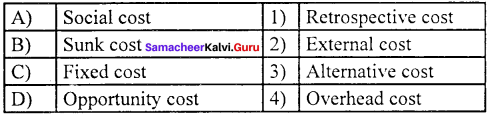(a) 2 3 4 1
(b) 2 1 4 3
(c) 4 3 2 1
(d) 2 4 1 3
(b) 2 1 4 3

Choose the correct statement

Question 5.
(a) In the long run, all the factors are fixed
(b) The LAC curve is called an envelope curve.
(c) LAC is equal to the long-run total cost
(d) LAC curve cannot be derived from short-run cost curves
(b) The LAC curve is called as an envelope curve.

Question 6.
(a) Revenue means the price of the product.
(b) Marginal revenue is equal to the price of the product.
(c) Revenue means sales revenue d. Average revenue is the total income of the firm.
(d) Average revenue is the total income of the firm
(c) Revenue means sales revenue d. Average revenue is the total income of the firm.

Choose the incorrect pair

Question 7.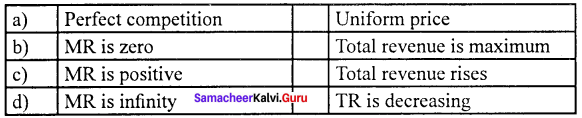(d) MR is infinity (iv) TR is decreasing

Question 8.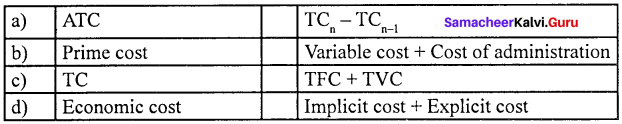(a) ATC  – (1) TCn – TCn-1

Analyze the reason for the following

Question 9.
Assertion (A) : If AR remains constant MR is also constant.
Reason (R) : MR is the addition made to the TR by the sale of an additional unit of a commodity.
(a) Both (A) and (R) are true, (R) is the correct explanation of (A)
(b) Both (A) and (R) are true, (R) is not the correct explanation of (A)
(c) Both (A) and (R) are false.
(d) (A) is true (R) is false.
(b) Both (A) and (R) are true, (R) is not the correct explanation of (A)

Question 10.
Assertion (A) : Real cost refers to the payment made to compensate the efforts and sacrifice of all factor owners.
Reason (R) : Adam Smith regarded pain and sacrifice of labour as real cost of production.
(a) Both (A) and (R) are true, (R) is the correct explanation of (A)
(b) Both (A) and (R) are true, (R) is not the correct explanation of (A)
(c) Both (A) and (R) are false.
(d) (A) is false (R) is true.
(a) Both (A) and (R) are true, (R) is the correct explanation of (A)

Choose the incorrect statement

Question 11.
(a) When AC is falling, MC lies below AC.
(b) When AC becomes constant, MC also equal to it.
(c) When AC starts increasing, MC lies above the AC.
(d) MC never cuts AC curve.
(d) MC never cuts AC curve.

Question 12.
(a) MR is equal to zero and TR is decreasing
(b) AR and MR curve depends upon the elasticity of AR curve
(c) When price elasticity is greater than one, MR is positive
(d) When price elasticity is less than one, MR is negative
(a) MR is equal to zero and TR is decreasing

Choose the odd one out

Question 13.
(a) Money cost
(b) Total variable
(c) Real cost
(d) prime cost
(b) Total variable

Question 14.
(a) When P = 3, Q = 8 then TR = 24
(b) When P = Q = 1 then TR = 10
(c) When P = 4, Q = 6 then TR = 27
(d) When P = 5, Q = 7 then TR = 35
(c) When P = 4, Q = 6 then TR = 27

Fill in the blanks with the suitable option given below

Question 15.
Economics profit is ______
(a) TR – TC
(b) TC – TR
(c) AC – MC
(d) None
(a) TR – TC

Question 16.
Cost function is the ________
(a) Relationship between total cost and output
(b) Relationship between revenue and cost
(c) Relationship between wages and interest
(d) None of the above
(a) Relationship between total cost and output

Question 17.
Break – even point is _________
(a) Total cost and total revenue
(b) Average revenue and financial revenue
(c) No profit – No loss point
(d) All the above
(c) No profit – No loss point

Choose the best option

Question 18.
Average fixed cost is obtained by
(a) TC / Q
(b) TVC / Q
(c) AC / Q
(d) TFC / Q
(d) TFC / Q

Question 19.
Long-run average cost curve can also be called as _____
(a) Planning curve
(b) Envelope curve
(c) Boat-shaped curve
(d) All the above
(d) All the above

Question 20.
Total fixed cost + Total variable cost is _____
(a) AC – MC
(b) TC
(c) TC – AC
(d) None
(b) TC

Part – B

Answer the following questions in one or two sentences

Question 1.
Give the definition for Economic Cost?

1. Economic cost refers to all payments made to the resources owned and purchased or hired by the firm in order to ensure their regular supply to the process of production. It is the summation of explicit and implicit costs.
2. Economic Cost is relevant to calculate the normal profit and thereby the economic profit of a firm.

Question 2.
What is the economic cost?
The economic cost is the summation of explicit and implicit costs.

Question 3.
What is the Average Variable Cost?
The average variable cost refers to the total variable cost per unit of output. It is obtained by dividing total variable cost (TVC) by the quantity of output [Q], AVC = TVC/Q, where AVC denotes Average variable cost, TVC denotes total variable cost and Q denotes the quantity of output.

Question 4.
What is a floating cost?
Floating cost refers to all expenses that are directly associated with business activities but not with asset creation.

Question 5.
What are variable costs?
Variable cost vary with the level of output. It is also called as prime cost, special cost or direct cost.

Question 6.
What is total revenue?
Total revenue is the amount of income received by the firm from the sale of its products.

Question 7.
What is marginal revenue?
Marginal revenue is the addition to the total revenue by the sale of an additional unit of a commodity.
MR = TRn – TRn-1

Part – C

Answer the following questions in One Paragraph

Question 1.
How can you calculate average fixed cost ?
Average fixed cost is the fixed cost per unit of output. It is obtained by dividing the total fixed cost by the quantity of output.
AFC = $$\frac { TFC }{ Q }$$
(Eg.) If TFC is 100;
Q = 10 Find AFC
AFC = $$\frac { TFC }{ Q }$$
= $$\frac { 1000 }{ 10 }$$
AFC = 100.

Question 2.
Define the Prime Cost?

1. All costs that vary with output, together with the cost of administration are known as Prime Cost.
2. Prime Cost = Variable Costs + Costs of Administration.

Question 3.
Write a note on average revenue.
AR = $$\frac { TR }{ Q }$$
AR = $$\frac { PQ }{ Q }$$ = P